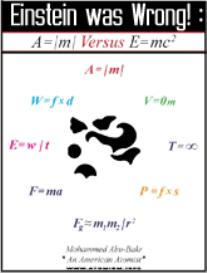# Einstein Was Wrong! : A = |m| Versus E = mc2

AIEB-1 In Stock
\$ 3.99 USD
Description Einstein Was Wrong! : A = |m| Versus E = mc2

This book explains how I arrived at my newly formulated mathematical formula A = |m| and how I use it to prove that Albert Einsteins famous mathematical formula E = mc2, which is derived directly from his special theory of relativity, is wrong. I show that it states a false equivalency between matter and energy. In the past, I used my two formulas P = f × s and E = w/t to do this, but now I find A = |m| a better and more forceful formula to use.

In addition, the book explains seven other formulas that I deem essential to providing correct mathematical descriptions of how the universe works. I formulated five of these. The other two were formulated back in 1687 by Sir Isaac Newton.